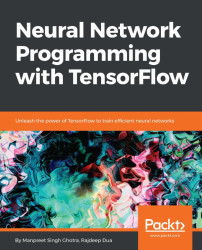•#### Neural Network Programming with Tensorflow#### Overview of this book

If you're aware of the buzz surrounding the terms such as "machine learning," "artificial intelligence," or "deep learning," you might know what neural networks are. Ever wondered how they help in solving complex computational problem efficiently, or how to train efficient neural networks? This book will teach you just that. You will start by getting a quick overview of the popular TensorFlow library and how it is used to train different neural networks. You will get a thorough understanding of the fundamentals and basic math for neural networks and why TensorFlow is a popular choice Then, you will proceed to implement a simple feed forward neural network. Next you will master optimization techniques and algorithms for neural networks using TensorFlow. Further, you will learn to implement some more complex types of neural networks such as convolutional neural networks, recurrent neural networks, and Deep Belief Networks. In the course of the book, you will be working on real-world datasets to get a hands-on understanding of neural network programming. You will also get to train generative models and will learn the applications of autoencoders. By the end of this book, you will have a fair understanding of how you can leverage the power of TensorFlow to train neural networks of varying complexities, without any hassle. While you are learning about various neural network implementations you will learn the underlying mathematics and linear algebra and how they map to the appropriate TensorFlow constructs.
Title PageCreditswww.PacktPub.comCustomer FeedbackPrefaceFree Chapter
Maths for Neural NetworksDeep Feedforward NetworksOptimization for Neural NetworksConvolutional Neural NetworksRecurrent Neural NetworksGenerative ModelsDeep Belief NetworkingAutoencodersResearch in Neural NetworksGetting started with TensorFlow## Understanding backpropagation

When a feedforward neural network is used to accept an input x and produce an output , information flows forward through the network elements. The input x provides the information that then propagates up to the hidden units at each layer and produces . This is called forward propagation. During training, forward propagation continues onward until it produces a scalar cost J(θ). The backpropagation algorithm, often called backprop, allows the information from the cost to then flow backward through the network in order to compute the gradient.

Computing an analytical expression for the gradient is straightforward, but numerically evaluating such an expression can be computationally expensive. The backpropagation algorithm does so using a simple and inexpensive procedure.

### Note

Backpropagation refers only to the method to compute the gradient, while another algorithm, such as stochastic gradient descent, refers to the actual mechanism.